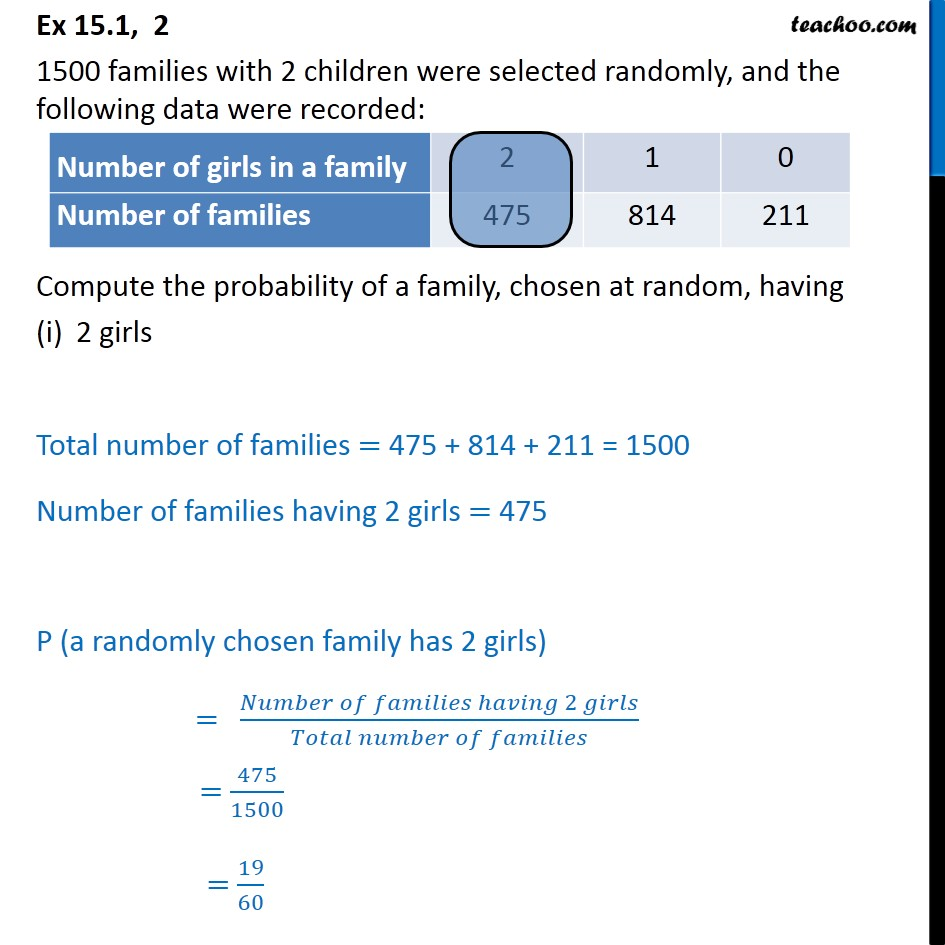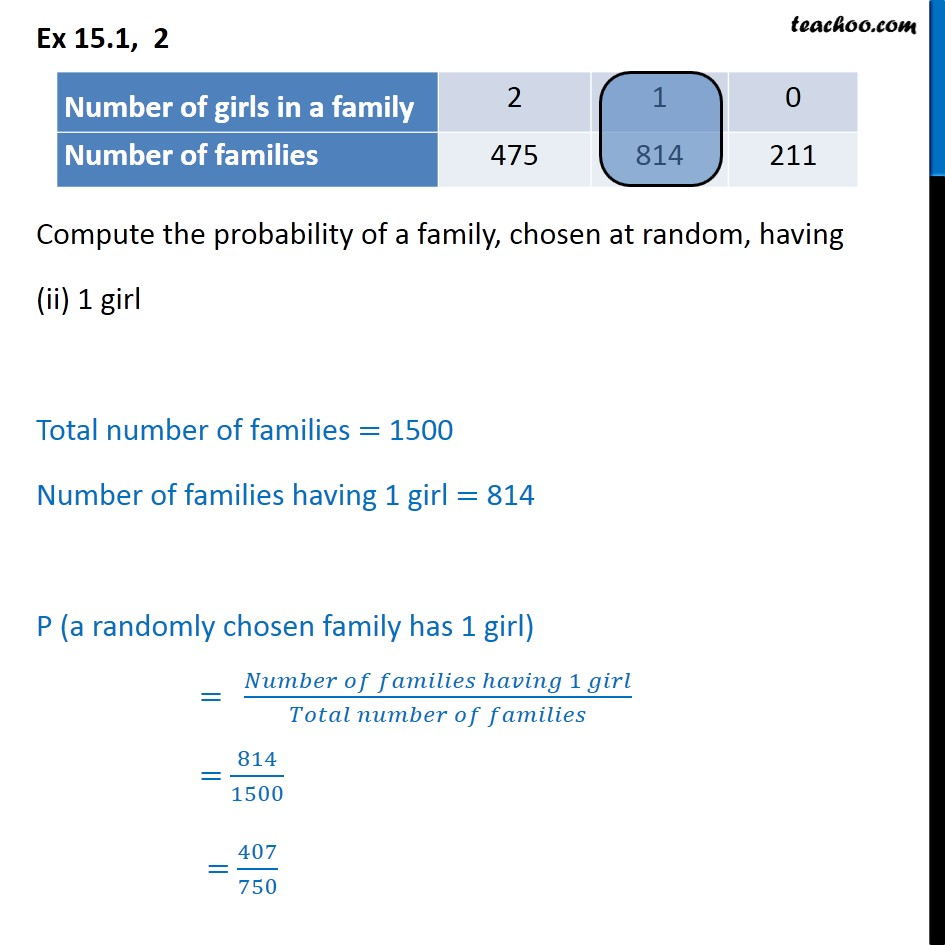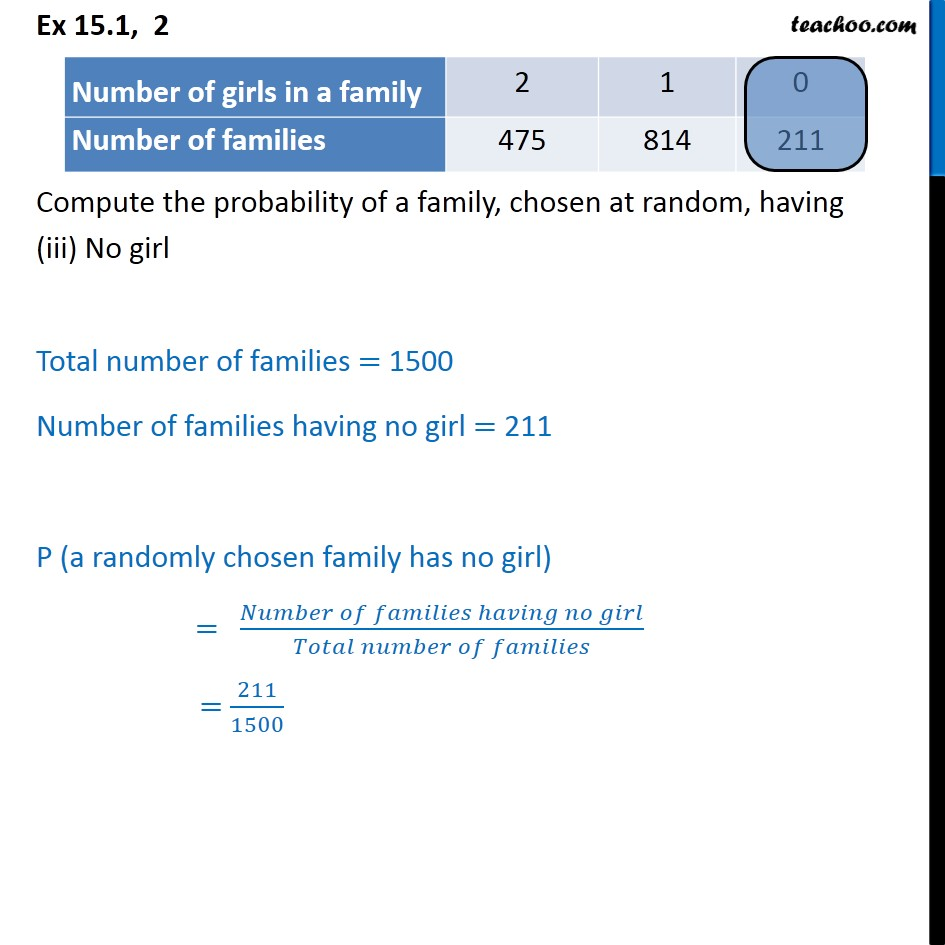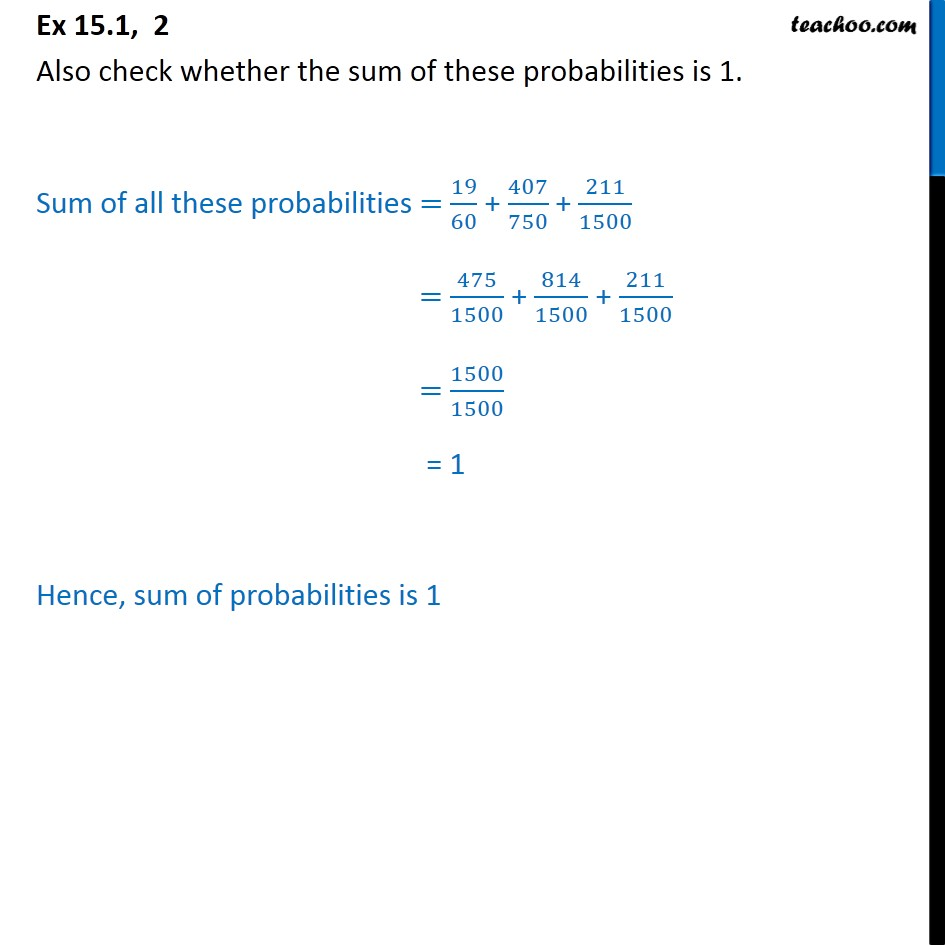Questions - Basic Probability

Probability
Serial order wise
• Questions - Basic Probability

• ExamplesLearn in your speed, with individual attention - Teachoo Maths 1-on-1 Class

### Transcript

Question 2 1500 families with 2 children were selected randomly, and the following data were recorded: Compute the probability of a family, chosen at random, having 2 girls Total number of families = 475 + 814 + 211 = 1500 Number of families having 2 girls = 475 P (a randomly chosen family has 2 girls) = 475/1500 = 19/60 Question 2 Compute the probability of a family, chosen at random, having (ii) 1 girl Total number of families = 1500 Number of families having 1 girl = 814 P (a randomly chosen family has 1 girl) = 814/1500 = 407/750 Question 2 Compute the probability of a family, chosen at random, having (iii) No girl Total number of families = 1500 Number of families having no girl = 211 P (a randomly chosen family has no girl) = 211/1500 Question 2 Also check whether the sum of these probabilities is 1. Sum of all these probabilities = 19/60 + 407/750 + 211/1500 = 475/1500 + 814/1500 + 211/1500 = 1500/1500 = 1 Hence, sum of probabilities is 1